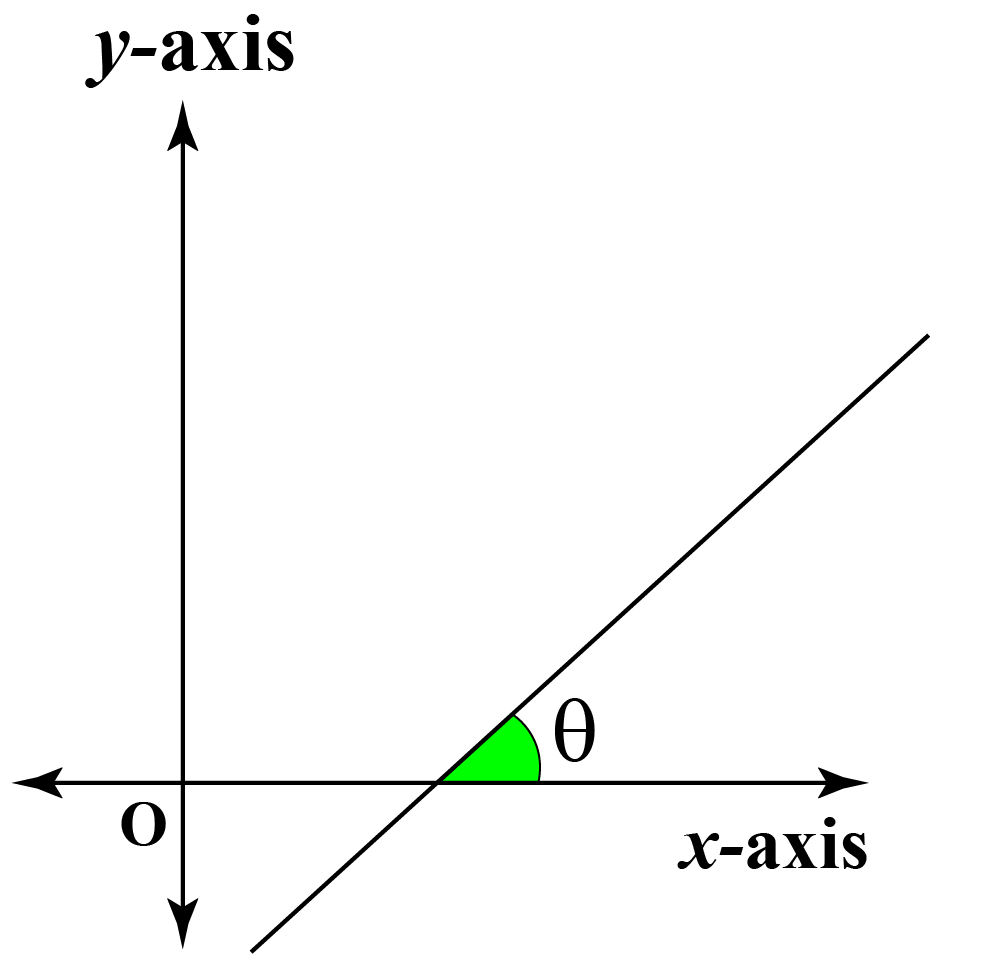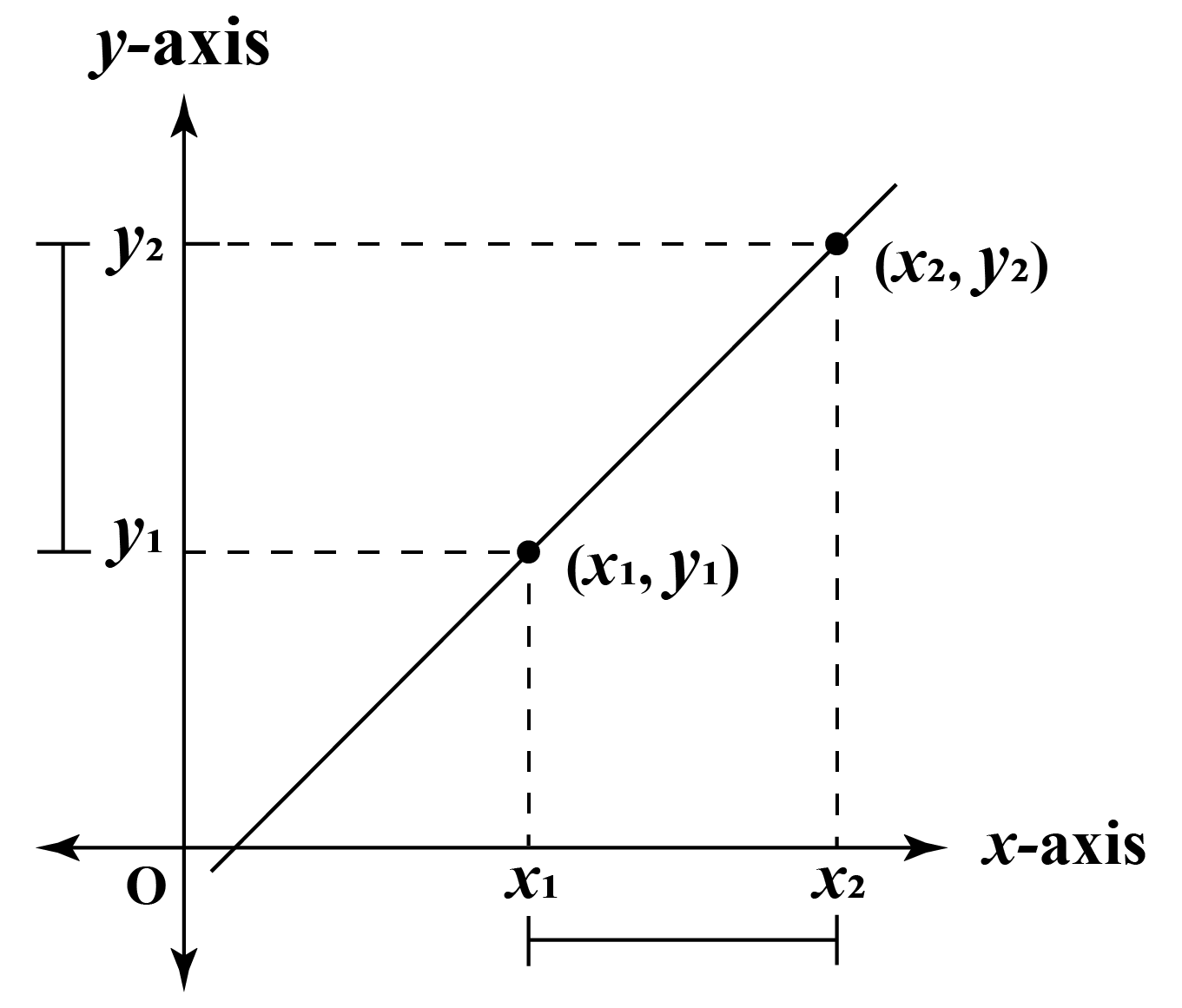In this mini-lesson, we shall explore the world of the gradient, by finding answers to questions like what is a gradient, what is a directional derivative, and understanding the properties of gradients with examples.

Let us understand how the gradient of line changes with a change in inclination of the line.

In this visualizer, move point B, and observe the change in the gradient of the line.

On moving the point B in the above simulation, the following three changes can be observed.

(a) coordinates of the point B
(b) the angle of the line
(c) the slope of the line

## Lesson Plan

 1 What Is the Definition of the Gradient? 2 Important Notes on Gradient 3 Think Tank 4 Solved Examples on Gradient 5 Interactive Questions on Gradient

## What Is the Definition of Gradient?

The gradient is the inclination of a line. The gradient is often referred to as the slope (m) of the line. The gradient or slope of a line inclined at an angle $$\theta$$ is equal to the tangent of the angle $$\theta$$.$m = tan\theta$

The gradient can be calculated geometrically for any two points $$(x_1, y_1)$$, $$(x_2, y_2)$$ on a line.$Slope =\frac{y_2 - y_1}{x_2 - x_1}$

Further, the gradient is a quantity, which helps to understand the variation in one quantity, with respect to another quantity.

For a function f(x) the gradient is calculated from its first derivative, $$\frac{d}{dx}.f(x)$$.

Summarizing the above sentences, we have:

$m = tan\theta = \frac{y_2 - y_1}{x_2 - x_1} = \frac{d}{dx}.f(x)$

Examples

1. The gradient of a line inclined at an angle of $$45^0$$ is $$m = tan 45^0 = 1$$
2. The gradient of a line joining the points(0, 3) and  (1, 5) is $$m = \frac{5 - 3}{1 - 0} = \frac{2}{1} = 2$$
3. The gradient of the tangent to the curve $$y = x^2 + x + 3$$ is $$\frac{d}{dx}.y = \frac{d}{dx}.(x^2 + x + 3)$$ =$$2x + 1 + 0 = 2x + 1$$
More Important Topics
More Important Topics